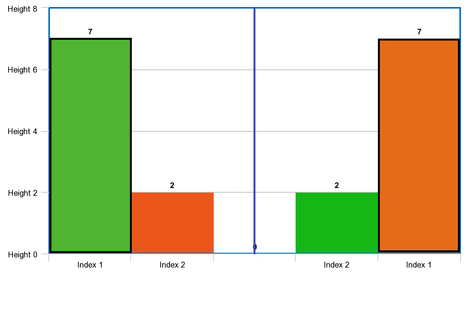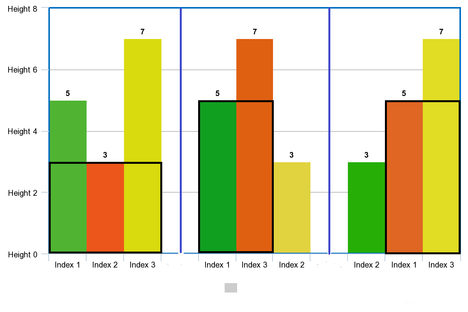# Histogram Reorderingcurly_bracesCriterion 2022 Round 19
Limits 1s, 512 MB

You are given an array of integers $H$, representing the height of the bars of a histogram. The width of each bar is 1. Now you will reorder the bars in such a way that the largest area of a rectangle in your histogram is the maximum possible amongst all possible reorderings.

You need to print a reordering of the histogram that satisfies the given condition. A reordering is a permutation of indexes from $1$ to $n$ where $n$ is the size of the array. If there are multiple possible answers, you have to print the lexicographically smallest permutation.

See the sample description for a better understanding.

## Input

Input starts with an integer $T( 1 \leq T \leq 10^5)$,

Each testcase starts with an integer $n( 1 \leq n \leq 10^5)$, the size of the array $H$.

The next line contains $n$ space-separated integers, $H_1, H_2, \dots, H_n(1 \leq H_i \leq 10^6)$

Sum of $n$ in all the test cases don’t exceed $10^6$.

## Output

For each testcase print $n$ integers in a line, the reordering of the histogram that satisfies the given condition.

## Sample

InputOutput
2
2
7 2
3
5 3 7

1 2
1 3 2


In test case 1:

There are two possible reorderings(shown in the picture, black rectangle indicates the largest rectangle)
If you use the ordering {1, 2}, the histogram stays {7, 2} which has a maximum rectangular area of 7 units.

If you use the ordering {2, 1}, the histogram becomes {2, 7} which also has a maximum rectangle of 7 units.Between the orderings, {1, 2} is lexicographically smaller.

In test case 2:

There are 6 possible reorderings(3 of those are shown in the picture), Among the orderings {1, 3, 2}, {3, 1, 2}, {2, 1, 3}, {2, 3, 1} all have a maximum rectangular area of 10 and the ordering {1, 3, 2} is the lexicographically smallest one.### Submit